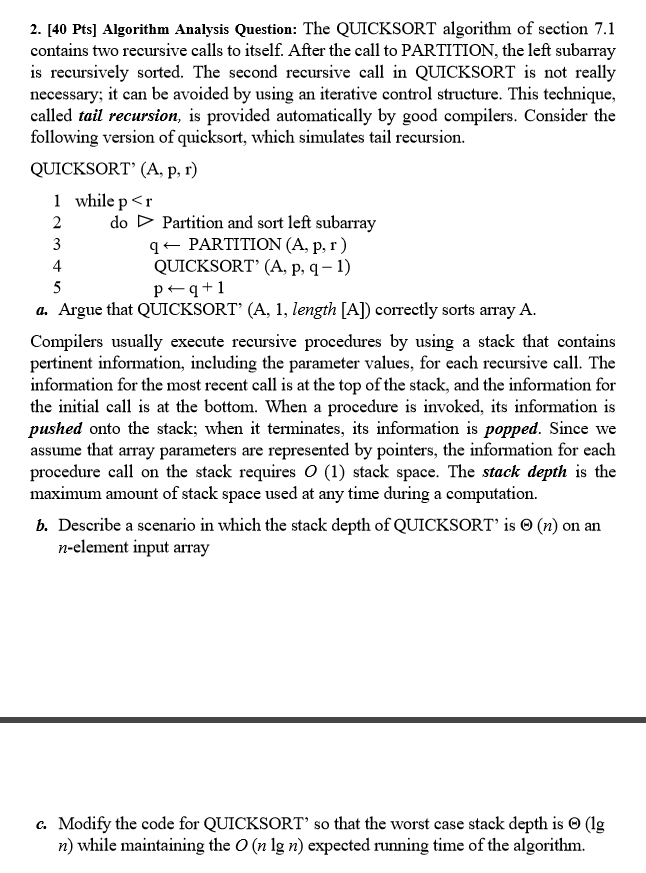# Answered! Algorithm Analysis Question:The QUICKSORT algorithm of section 7.1 contains two recursive calls to itself. After…Algorithm Analysis Question:The QUICKSORT algorithm of section 7.1 contains two recursive calls to itself. After the call to PARTITION, the left subarray is recursively sorted. The second recursive call in QUICKSORT is not really necessary:it can be avoided by using an iterative control structure. This technique, called tail recursion, is provided automatically by good compilers. Consider the following version of quicksort, which simulates tail recursion. QUICKSORT’ (A, p, r) while p

a) We should start with what is tail recursion ! In a recursive function if recursive call is the last thing executed by the function then it is tail recursive. As we can see here the function only makes one recursive call and that to sort the the left array. After recursive calling of the QUICKSORT(A,p, q -1), we enter in to the left and then it goes on and goes. Sorting the left part of each array, but how is right array sorted? the statement p–> q +1 takes care of that, as p is kept to have q+1 then next time we enter the loop the right array is sorted becasue q <– partition(A, p, r), here p has been changed to the starting index of right array and r is the ending index. Hence, both the arrays are taken case of. The benefit of this would be using the stack frame again, meaning a tail-recursive function will only use a single stack frame rather than n stack frame as by normal recursive function.

b) We managed to reduce the number of recursive calls by using tail-recursion but it can still use O(n) space in the worst case. How? If the array is sorted in decreasing order and last element is our pivot, then out left array will always have n-1 elements, making the stack go up to n. So this can be the scenario.

Don't use plagiarized sources. Get Your Custom Essay on
Answered! Algorithm Analysis Question:The QUICKSORT algorithm of section 7.1 contains two recursive calls to itself. After…
GET AN ESSAY WRITTEN FOR YOU FROM AS LOW AS \$13/PAGE

c) What if we just called recursion for the smaller part after the partition happens? If that is done, we can always have O(log n) as stack depth. Consider the code:
QuickSort(A, p, r)
while (p < r)
q = partition(A, p, r)
if (q – p < r – q) // if left part is smaller
quickSort(A, p, q – 1) //recursive call for left part
p = q + 1 //handle the right part
else //if right part is smaller
quickSort(A, q + 1, r) // recursive call for right part
r = q – 1 //handle the left part

This will ensure that recursion is always done on smaller part of the array i.e. reducing the size of the stack to almost half. The runtime is not affected as the same amount of work has to be done as before.

If you have any further doubts, let me know in the comments !